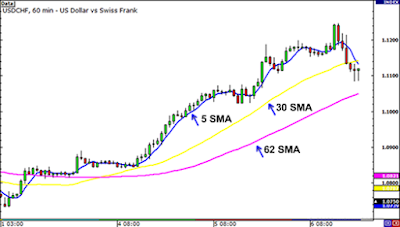# simple moving average (sma) explanation

The simple moving average (SMA) is the simplest type of MA in forex analysis.

SMA is calculated by adding the last period “X” to the closing price and dividing by “X”.

Confused?

Here's the explanation.

### Calculating Simple Moving Average (SMA)

When placing a plot of 5 period SMA on a 1-hour chart, we automatically add up the closing price of the last 5 hour range then divide by 5.

And will get the average closing price in the last five hours.  Next we just need to line up all those average closing prices.

If you want to place a 5 period SMA on a 10-minute chart, you just need to add up the closing price from the last 50 minutes and then divide by 5.

Likewise, if you want to place a 5 period SMA on a 30-minute chart, you only need to add the closing price from the last 150 minutes and then divide by 5.

However, you don't need to bother calculating this SMA because it will be calculated automatically by the trading platform.

The reason to tell you is because it is very important to understand about this indicator, so you can also know the formula for editing and changing this indicator.

Understanding how analytical indicators work will help you use them according to your needs and changing market conditions.

Like most other indicators, the simple moving average has a delay in operation.

Since you're taking an average of historical prices, you're actually only looking at past price movements and forecasts of future prices.

The following is an example of how a simple moving average smoothes price movements.In the chart above there are 3 SMAs in 1 hour period for USD/CHF and as you can see, the bigger the current period, the bigger the price lag.

Notice the 62 SMA is far below the 30 SMA and 5 SMA.  This happens because the 62 SMA is obtained from the closing price of 62 periods divided by 62.

So in conclusion, the longer the SMA period, the slower the reaction to price movements.

The SMA on this chart shows the overall market sentiment at the time.  Here, we can see that the currency pair is trending.

Instead of just looking at the current market price, the MA gives us a broader view, as well as measuring the direction of the price trend in the future.

But there is one drawback to the simple moving average, which is that it sometimes gives false signals during price spikes.

In the next lesson, we will describe other types of MA to avoid this kind of problem.